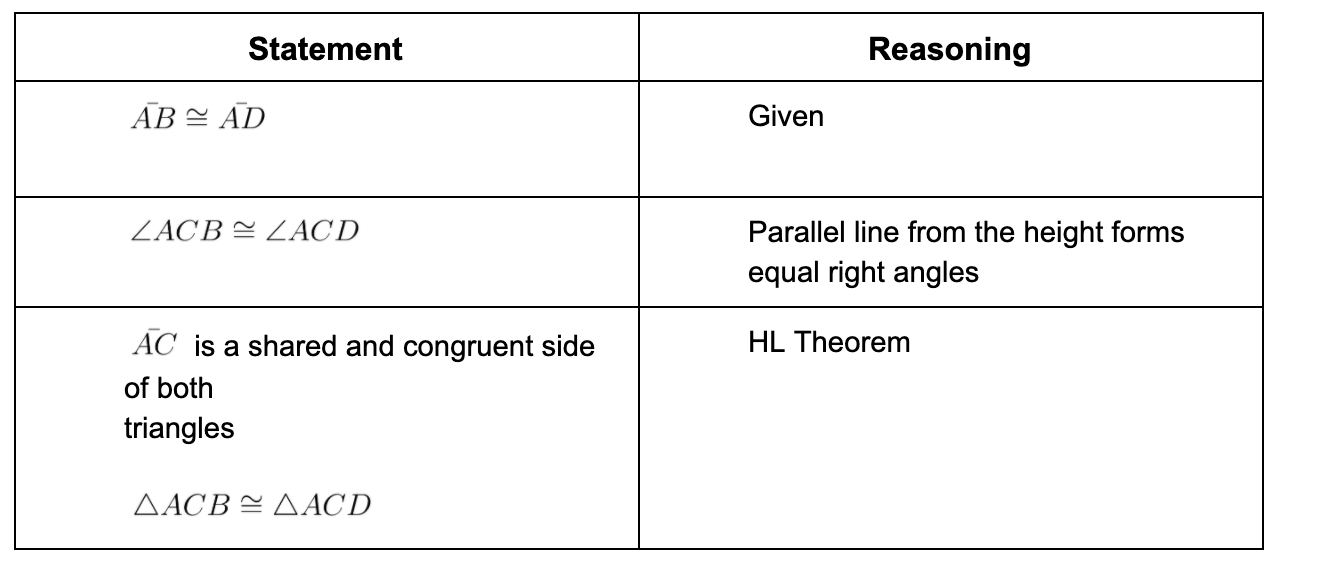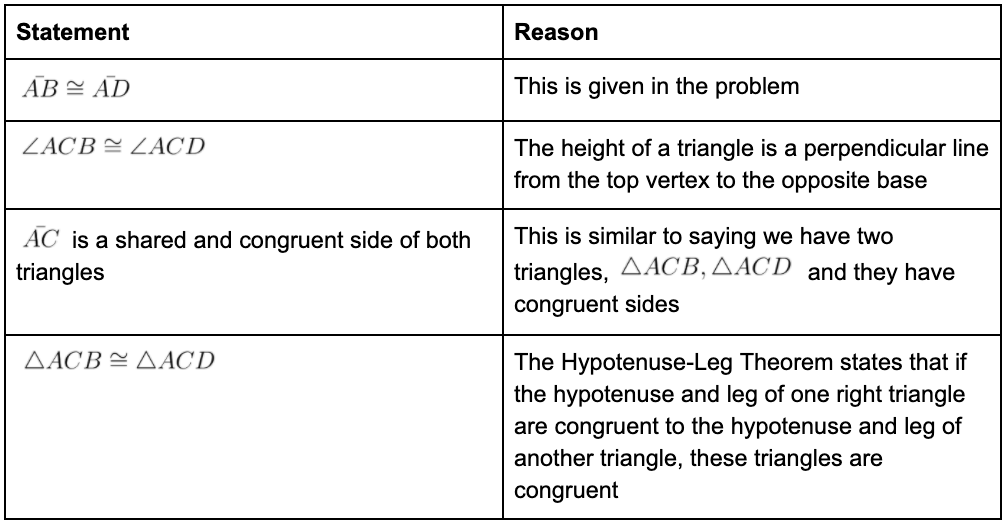# Common Core: High School - Geometry : Triangle Proofs

## Example Questions

### Example Question #1 : Triangle Proofs

Are the following two triangles congruent?  If so, what theorem can we use to prove this to be true?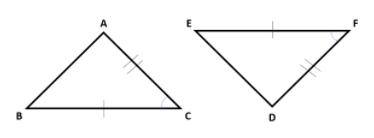Yes, they are congruent by the SAS Theorem

There is not enough information to answer this question

Yes, they are congruent by the ASA Theorem

No, they are not congruent

Yes, they are congruent by the SAS Theorem

Explanation:

From the figure, we see that there are two congruent pairs of corresponding sides,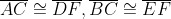and one congruent pair of corresponding angles,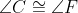The Side-Angle-Side Theorem (SAS) states that if two sides and the angle between those two sides of a triangle are equal to two sides and the angle between those sides of another triangle, then these two triangles are congruent.

### Example Question #1 : Triangle Proofs

Which of the following theorems prove that the following two triangles are congruent?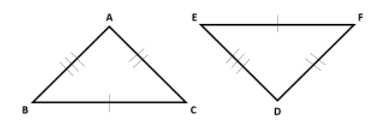Side-Angle-Side Theorem

Angle-Side-Angle Theorem

Angle-Angle-Side Theorem

Side-Side-Side Theorem

Side-Side-Side Theorem

Explanation:

These two triangles share three corresponding congruent sides.  The Side-Side-Side Theorem (SSS) states that if the three sides of one triangle are congruent to their corresponding sides of another triangle, then these two triangles are congruent.

### Example Question #132 : Congruence

Which of the following pairs of triangles are congruent by the ASA Theorem?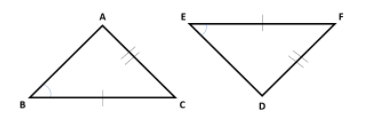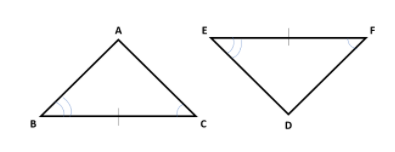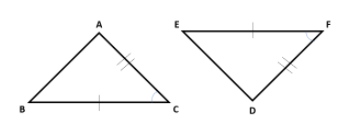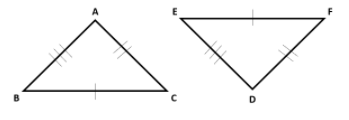Explanation:

The Angle-Side-Angle Theorem (ASA) states that if two angles and their included side are congruent to two angles and their included side to another triangle, then these two triangles are congruent.  Our first option cannot be correct because this figure does not give any information about the angles.  This could be proven using the SSS Theorem.  The second figure only gives information about one angle, this could be proven using the SAS Theorem.  The third option again only gives information about one angle.  The fourth figure has two angles congruent, and their included side congruent.  Clearly this is the only figure that could have congruent triangles proven through the ASA Theorem.

### Example Question #1 : Triangle Proofs

Which of the following theorems would prove that the following two triangles are similar?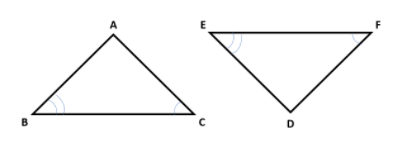There is not enough information to determine these triangles are similar.

SAS Theorem

AA Theorem

SSS Theorem

AA Theorem

Explanation:

When we look at this figure we see that we have two pairs of congruent corresponding angles,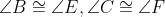. The Angle-Angle Theorem (AA) states that if two angles of one triangle are congruent to two angles of another triangle, then these triangles are similar.

### Example Question #134 : Congruence

Are the following two triangles similar?  If so, which theorem proves this to be true?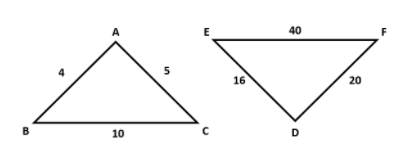No, these triangles are not similar

Yes, these triangles are similar by the SSS Similarity Theorem

Yes, these triangles are similar by the AA Similarity Theorem

There is not enough information to determine if these triangles are similar or not

Yes, these triangles are similar by the SSS Similarity Theorem

Explanation:

The Side-Side-Side Similarity Theorem states that if all three sides of one triangle are proportional to another, then these triangles are similar.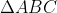and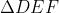are similar by this theorem because each of their sides are proportional by a factor of 4.

### Example Question #1 : Triangle Proofs

True or False: Side-Side-Angle is a proven theorem to prove triangle congruence.

False

True

False

Explanation:

Just because a triangle has two sides and one angle congruent to the two sides and angle of another triangle does not guarantee these two triangles’ congruence.  For the two triangles to be congruent, the two sides that are congruent must contain the congruent angle as well.  Below are two triangles that share congruent sides and one angle, but are not congruent.### Example Question #2 : Triangle Proofs

Prove that the parallelogram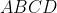has two congruent triangles,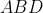and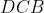.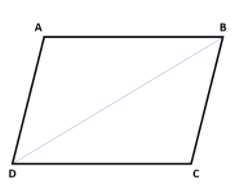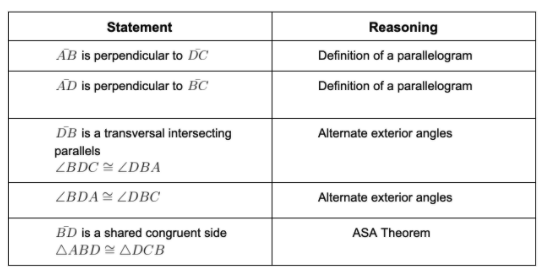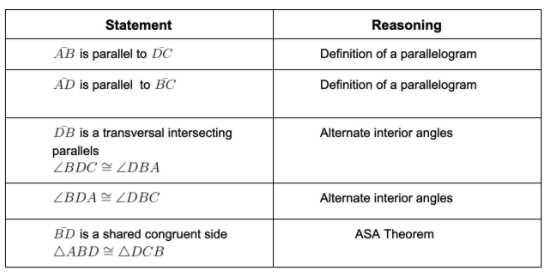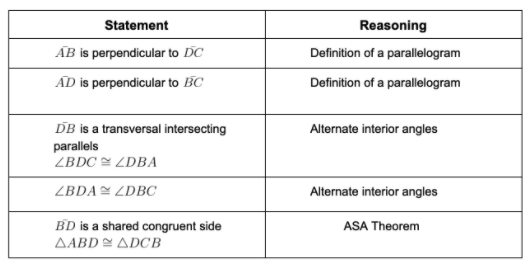Explanation: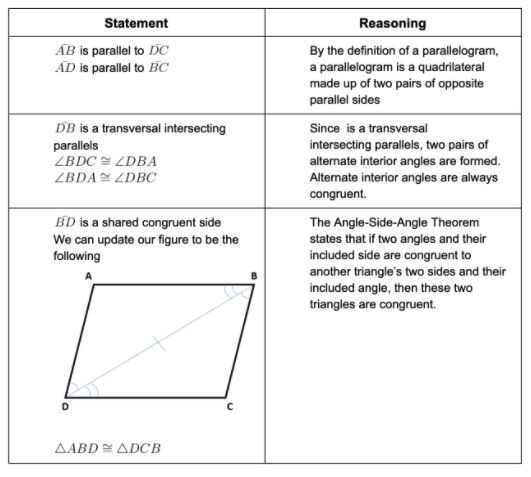### Example Question #136 : Congruence

Assume that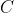is the midpoint of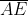and the midpoint of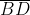. Prove that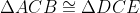.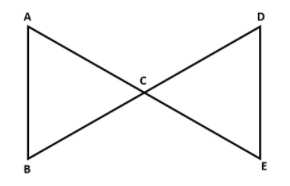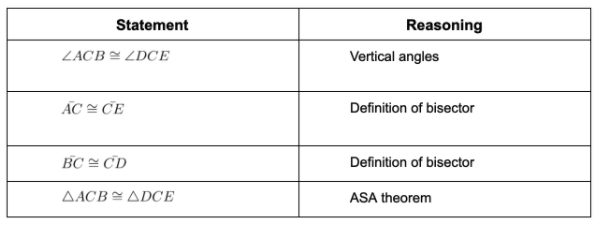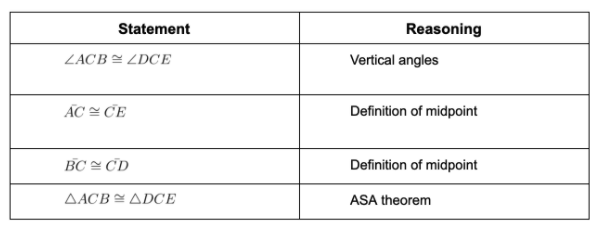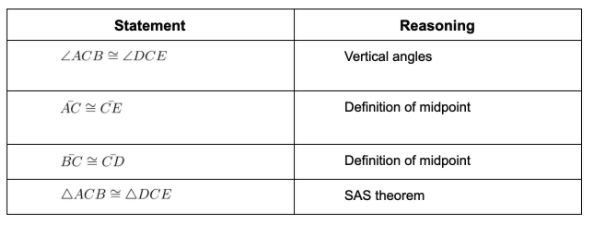Explanation: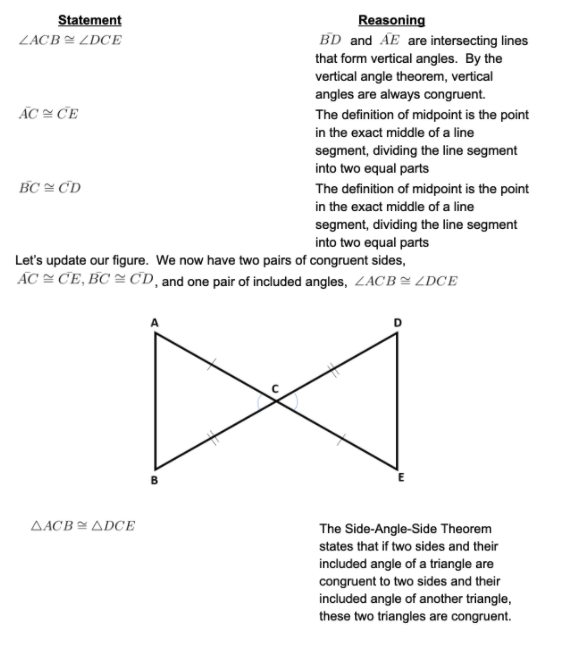### Example Question #1 : Triangle Proofs

Which of the following describes the Angle-Side-Angle Similarity Theorem?

If two triangles share two pairs of congruent angles, then these two triangles are similar

If two triangles have two pairs of sides are of the same proportions and their included angles are congruent, then these two triangles are congruent

If two triangles have two pairs of sides are of the same proportions and their included angles are congruent, then these two triangles are similar

If two triangles share all three pairs of sides in the same proportions, then these two triangles are similar

If two triangles have two pairs of sides are of the same proportions and their included angles are congruent, then these two triangles are similar

Explanation:

The Angle-Side-Angle Similarity Theorem states that if two triangles have two pairs of sides are of the same proportions and their included angles are congruent, then these two triangles are similar.  To be similar triangles can be different sizes, but all angles must be congruent.  If two triangles have a pair of congruent angles, then we know their opposite side of that angle must be in proportion to each other.  If we also have two pairs of sides that are of the same proportions then these triangles would be similar.  This theorem guarantees these conditions are met.

### Example Question #1 : Triangle Proofs

Consider trianglebelow.  Line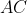is the height of the triangle.  Prove that triangleis made up of two congruent triangles,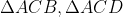.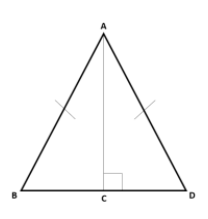Proof: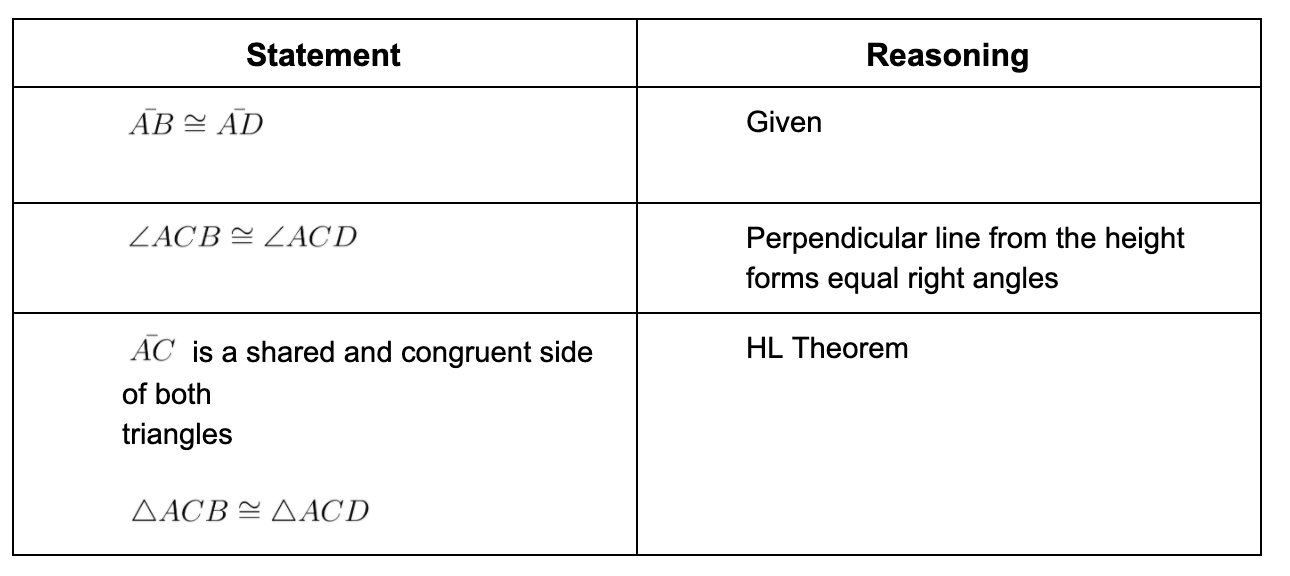Proof: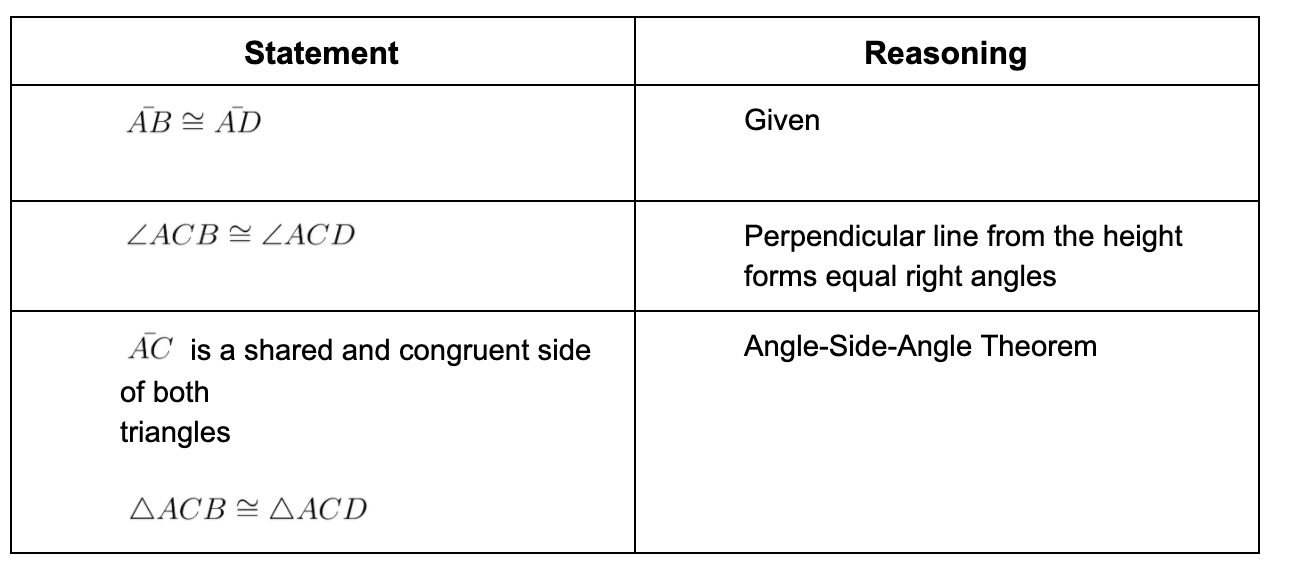Proof: